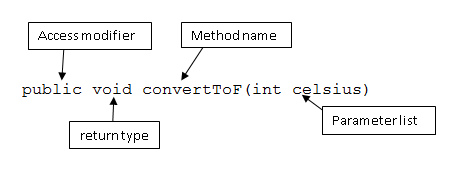← Home

# Methods

Here's an example of a method:

``````1public static void convertToF(int celsius) {
2    int fahrenheit = ((celsius * 9) /5) + 32;
3    System.out.println("C:" + celsius + " F: " + fahrenheit);
4}
``````

Don't worry if you don't quite understand what's going on in the code above for now. What you are looking at, however, is a basic method which converts a temperature you specify in degrees Celsius to its equivalent in Fahrenheit.

## Creating and defining your very first method

Before creating your first method, there are a few things you need to know. Mainly, the two ingredients needed to create a method: the method header and the method body.

To do this, let's first decipher the method above.

The very first line of the method named `convertToF` is known as the method header.

``````1public void convertToF(int celsius)
``````

When we refer to a method body, we basically mean everything within the curly brackets after a method header.

``````1public void convertToF(int celsius) {
2    int fahrenheit = celsius * 9/5 + 32;
3    System.out.println(" C:" + celsius + " F: " + fahrenheit);
4}
``````

A method header consists of the following:Sometimes, we want methods to 'return' something to us, and we specify this in the return type. For example, you might want one of your employees to give you a report. In this case, however, you have specified that you don't want anything. Thus, you have told your employee (this method) to return `void` i.e. nothing.

NOTE: This can be changed to other return types of your such as `int`, `String`, `boolean`. However, for now, focus on setting methods to return `void`.

Following the return type is the method name, in this case `convertToF`. This is something you specify too. By convention, method names start with a lowercase letter and every other word after starts with a capital. Try to make method names reasonably short and meaningful as to what task they carry out, as this will make it easier in the long run.

Following the method name comes the parameter list (the contents of the brackets). This enables you to pass values into a method. For example, in the `convertToF` method, it accepts one parameter (`celsius`) which is declared to be of type int. This means that when using this method later on, you can input something like `convertToF(20)` in order to convert 20 degrees Celsius to Fahrenheit.

For now, you are going to have to accept that there is something called an access modifier that will be at the start of every method you write. In this case, we will always be using the access modifier `public`. For the curious, access modifiers are related to classes (a key feature of an object-orientated language like Java), and other 'access modifiers', apart from public, exist, such as `private` and `protected`. It is also not actually a requirement to have an access modifier at the beginning of a method.

## Static methods

Hitherto you've only met static methods, which are methods that belong to a class, as opposed to an instance of a class. For instance, the method `Integer.parseInt()` (which is in the next part) is a static method. If I, however, create an integer like so:

``````1Integer age = 5;
``````

`age` does not have the method `.parseInt()`. What I'm trying to say is that when you meet many of these methods the bit after the full stop is a static method. Similarly, the methods you've created and will create with the `static` keyword are also static methods. If you were to remove the `static` keyword from them they would not be able to be called by the `main` method.

N.B.: `Math.abs()`, and `Math.sqrt()` are also statics, as are other methods inside the `Math` package, such as trigonometric functions `Math.sin()`, `Math.cos()`, and `Math.tan()`.

## Calling methods

To call the method from the `main` method or any other non-static method, write:

``````1public static void main(String[] args) {
2    int converted = convertToF(10);
3    System.out.println(converted);
4}
``````

### Ex 1

Write a method called `pizzazz` which accepts one integer and displays `Pizzazz` on the terminal if the integer is fully divisible by 7. If the integer isn't divisible by 7, display `A tragic number has been entered` instead.

### Ex 2

Write a method called `checkEvenOdd` which accepts one integer and checks if it is odd or even. If the integer is odd, `The number is odd` will be displayed on the terminal.

### Ex 3

Write a method called `findTriangleArea` which accepts two parameters, the base length and height of a triangle, then calculates and displays the area of the triangle. Hint: As if you didn't know - the area of a triangle = 0.5 base height

### Ex 4

Write a method called `findParaArea` which accepts two parameters, the base length and height of a parallelogram, then calculates and displays its area on screen. Hint: the area of a parallelogram = base * height

### Ex 5

Copy & paste the method for Ex 3 and rename it `findTrapeArea`. The method should now calculate the area of a given trapezium. What changes should you make? Hint: the area of a trapezium (top length + bottom length)/2 * height

Some pre-determined methods →